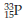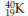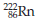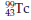×
Get Full Access to Introductory Chemistry - 5 Edition - Chapter 4 - Problem 98p
Get Full Access to Introductory Chemistry - 5 Edition - Chapter 4 - Problem 98p

×

# Determine the number of protons and neutrons in each isotope.(a) (b) (c) (d)ISBN: 9780321910295 34

## Solution for problem 98P Chapter 4

Introductory Chemistry | 5th Edition

• Textbook Solutions
• 2901 Step-by-step solutions solved by professors and subject experts
• Get 24/7 help from StudySoup virtual teaching assistantsIntroductory Chemistry | 5th Edition

4 5 1 426 Reviews
30
1
Problem 98P

Problem 98P

Determine the number of protons and neutrons in each isotope.

(a)(b)(c)(d)Step-by-Step Solution:

Solution 98P

Step 1:

The isotopes of the same element have similar chemical behavior.

(a) In 1535P the atomic number is 15, so there are 15 protons and 15 electrons. The mass  number     is 35, so there are 10 (=35-15) neutrons.

Step 2 of 4

Step 3 of 4

##### ISBN: 9780321910295

Unlock Textbook Solution